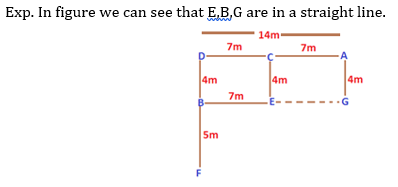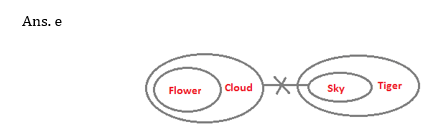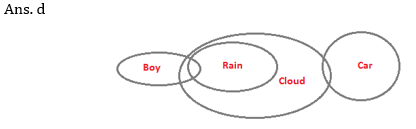# SBI PO Pre Reasoning Ability Quiz – 12

## SBI PO Pre Reasoning Ability Quiz 2022

Almost all major competitive exams in the country have a section on Reasoning Ability, and it is also one of the most scoring sections. Candidates can ace this section in exams if they practice regularly in a dedicated manner. So here we are providing you with the SBI PO Pre Reasoning Ability Quiz to help you prepare better. This SBI PO Pre Reasoning Ability Quiz includes all of the most recent pattern-based questions, as well as Previous Year Questions. This SBI PO Pre Reasoning Ability Quiz is totally FREE. Candidates will be provided with a detailed explanation of each question in this SBI PO Pre Reasoning Ability Quiz. Candidates must practice this SBI PO Pre Reasoning Ability Quiz to achieve a good score in the Reasoning Ability Section.

Directions (1-3): Study the following information carefully and answer the given questions.
Point D is 14 m towards the West of Point A. Point B is 4 m towards the South of point D. Point F is 9 m towards the South of point D. Point E is 7 m towards the East of point B. Point C is 4 m towards the North of point E. Point G is 4 m towards the South of point A.
1. Which of the following points are in a straight line?
(a) D, E, A
(b) E, G, C
(c) D, B, G
(d) E, B, G
(e) F, B, C

Ans. d2. A is in which direction with respect to C?
(a) East
(b) West
(c) North
(d) South
(e) Cannot be determined

Ans. a3. If a person walks 5 m towards North from point F and then takes a right turn, which of the following points would he reach first?
(a) G
(b) D
(c) E
(d) A
(e) C

Ans. cDirections (4-6): Study the following information carefully to answer these questions.
Ten executives A, B, C, D, E, F, G, H, I and J stay in flats in two rows which are facing to each other. One row has 5 flats facing North and the other row has 5 flats facing south. F’s flat is second to the right of J’s flat, which is exactly in front of C’s flat facing North. D’s flat is on the immediate left of J’s flat and A’s flat is on the immediate left of C’s flat B’s flat is on the right of C’s flat. I and E have flats at the two ends of the same row. Flats E and H are opposite to each other.
4. Which of the following is the correct position of G’s flat?
(a) Opposite to B’s flat
(b) Opposite to C’s flat
(c) To the left of F’s flat
(d) To the left of J’s flat
(e) None of these

Ans. c5. Who stays opposite to B ?
(a) F
(b) G
(c) D
(d) F or G
(e) None of these

Ans. c6. Which of the following statements is definitely true?
(a) J’s flat is facing North.
(b) G and A have flats opposite to each other.
(c) B’s flat is to the right of E’s flat.
(d) G’s flat is to the left of J’s flat.
(e) None of these

Ans. bDirection (7-10): In each question below are given three statements followed by four conclusions numbered I, II, III and IV. You have to take the given statements to be true even if they seem to be at variance with commonly known facts. Read all the conclusions and then decide which of the given conclusions logically follows from the given statements, disregarding commonly known facts:

7. Statements: a. All flowers are clouds.
b. No cloud is sky.
c. All sky are tigers.
Conclusions: I. Some clouds are flowers.
II. All clouds are flowers.
III. Some tigers are sky.
IV. All tigers are sky.
(a) Only II and IV follow
(b) Only either I or II follows
(c) Only either III or IV follows
(d) All follow
(e) None of these8. Statements: a. Some dogs are rats.
b. All rats are trees.
c. Some trees are not dogs.
Conclusions: I. Some trees are dogs.
II. All dogs are trees.
III. All rats are dogs.
IV. All trees are dogs.
(a) None follows
(b) Only I follows
(c) Only I and II follow
(d) Only II and III follow
(e) All follow9. Statements: a. Some boys are rains.
b. All rains are clouds.
c. Some clouds are cars.
Conclusions: I. Some clouds are boys.
II. Some cars are boys.
III. Some cars are rains.
IV. Some rains are boys.
(a) None follows
(b) Only IV follows
(c) Only I follows
(d) Both I and IV follow
(e) All follow10. Statements: a. All bricks are flowers.
b. Some houses are flowers.
c. All pens are houses.
Conclusions: I. Some houses are bricks.
II. Some pens are flowers.
III. Some flowers are bricks.
IV. No pen is flower.
(a) Only either II or IV and III follow
(b) Only either II or IV and I follow
(c) Only either I or II and IV follow
(d) None follows
(e) All follow